Translation Worksheets

Translation worksheets contain a variety of practice pages to translate a point and translate shapes according to the given rules and directions. Also, graph the image and find the new coordinates of the vertices of the translated figure in these sheets.

Printing Help - Please do not print translation worksheets directly from the browser. Kindly download them and print.

Translation of a point

Translate the given point up, down, left or right based on the given directions. Each worksheet has 12 problems.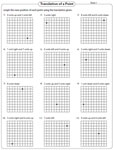Choose the correct translation

In these translation worksheets choose the image obtained after translating the point as described, from the given multiple choices.Translate the shapes

Translate the shape following the instructions given above each problem. Each worksheet has nine problems for practice.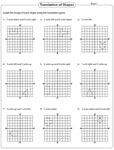Translate the triangles

Translate each triangle on the grid. Translate the triangles to the left, right, up or down.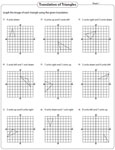Translate each quadrilateral in the grid following the given instructions. Graph and label the translated image.Write the rules

Write directions to describe each translation under each figure. Ex. Translate 2 units up and 3 units right.Writing coordinates: With graph

In these worksheets graph the image of the given shape after translation and find the coordinates of the vertices of the image.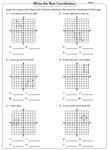Writing New coordinates

The coordinates of a figure are given. Write the new coordinates of the image obtained after the given translation.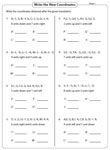The whole batch of translation worksheets can be downloaded instantly.

Related Worksheets